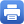Columbia Home
Algebraic Geometry and Number Theory

Select Commutative Algebra in the fall semester, and then pick a specialization in the spring; either Algebraic Geometry or Algebraic Number Theory.

### Commutative Algebra

I Basic notions for rings and modules

• Rings, ideals, modules
• Localization
• Primary decomposition
• Integrality
• Noetherian and Artinian Rings
• Noether normalization and Nullstellensatz
• Discrete valuation rings, Dedekind domains and curves
• Dimension theory, Hilbert functions, Regularity
• Sheaves and affine schemes

### Algebraic Geometry

I Varieties

• Projective Varieties
• Morphisms and Rational Maps
• Nonsingular Varieties
• Intersections of Varieties

II Schemes

• Basic properties of schemes
• Separated and proper morphisms
• Quasi-coherent sheaves
• Weil and Cartier divisors, line bundles and ampleness
• Differentials
• Sheaf cohomology

III Curves

• Residues and duality
• Riemann-Roch
• Branched coverings
• Projective embeddings
• Canonical curves and Clifford’s Theorem

### Algebraic Number Theory

• Local fields
• Global fields
• Valuations
• Weak approximation
• Chinese Remainder Theorem
• Ideal class groups
• Minkowski’s theorem and Dirichlet’s unit theorem
• Finiteness of class numbers
• Ramification, different and discriminantsPrint this page